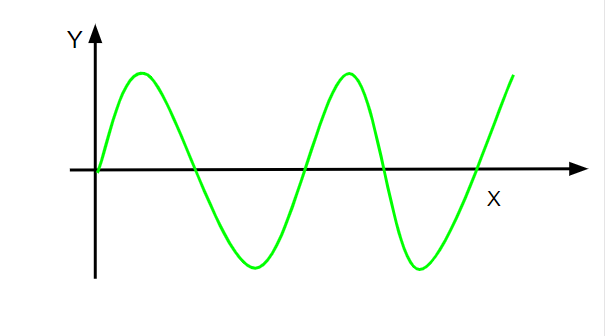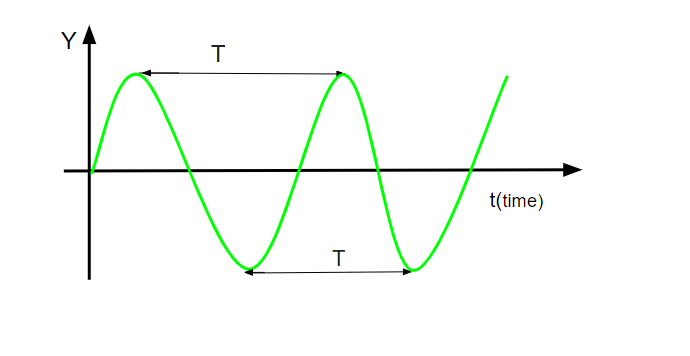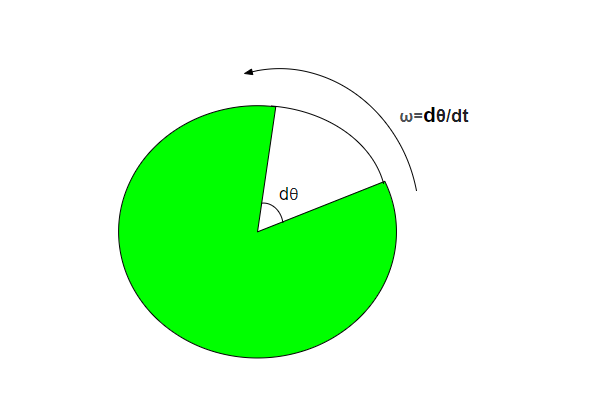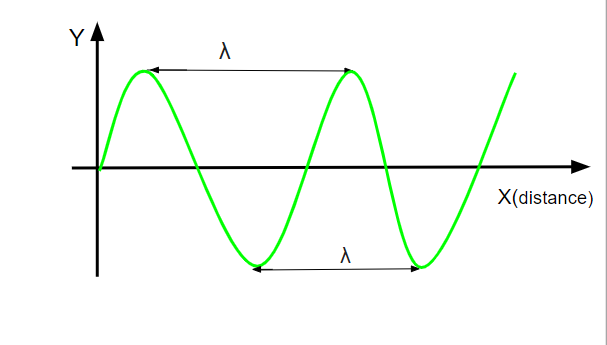# Wavelength To Frequency Formula

• Last Updated : 03 Mar, 2022

Frequency is defined as the number of cycles completed per unit time. “OR”. It can also be defined as the number of oscillations per unit time.  SI unit of frequency is Hertz (Hz). It is denoted by ‘f’.Dimensions of frequency = [M0L0T-1]

Terms to remember

• Time period(T): The time taken to complete one oscillation is called a time period. It is denoted by ‘T’. The SI unit of time period is “second(s)”. Dimension of time period = [M0L0T1].• Oscillation: The process of moving back and forth or up and down regularly after a fixed interval of time is called oscillation.
• Wave speed(v): The speed at which a wave travels is called wave speed. It is denoted by “v”. The SI unit of wave speed is “meter per second“.
• Angular frequency(ω): It is defined as the angular displacement per unit time. It is denoted by “ω”. The SI unit of angular frequency is “rad/sec“. Dimension of angular frequency = [M0L0T-1].• Wavelength(λ): The distance between corresponding points of two consecutive waves is called wavelength. Its symbol is “λ”. SI unit of wavelength is “meter(m)”. Dimension of wavelength = [M0L1T0].### Wavelength to Frequency formulae

We know that speed is the distance over time.

speed = distance/ time

v = λ/T

v = λ × 1/T

v = λf ⇢ [1/T- frequency(f)]

f = v/λ

v- speed

λ- wavelength

T- time period

f- frequency

ω- angular frequency

Formula to remember

• f = 1/T ⇢ ( T- time period)
• f = v/λ ⇢ ( v- wave speed and λ – wavelength)
• f = ω/2π ⇢  (ω – angular frequency)

Applications of Frequency

• Used for Ship and Aircraft communication
• Used for disaster prevention
• Used for Police and Fire radio communication
• Low frequency is used for the detection of deep defects
• Low frequency is used for AM broadcasting in Europe

### Sample Problems

Question 1: Find the frequency of a wave where one cycle is completed in 0.008 sec.

Solution:

Time (T) = 0.008 sec

Frequency = 1/time period

f= 1/t

f = 1/0.008

f = 125 Hz

Therefore, frequency will be 125 Hz

Question 2: The wavelength of a light wave is 50nm. Find the frequency of the light waves.

Solution:

Wavelength(λ) = 50nm = 50 × 10-9m = 5 × 10-8m

Speed of light (v) = 3 × 108m/s

f = v/λ

f = (3 × 108)/ (5 × 10-8)

f = 0.6 × 1016Hz

Therefore, frequency will be 0.6 × 1016Hz.

Question 3: Find the wavelength of a sound wave traveling at the speed of 350 m/s has a frequency of 1800Hz.

Solution:

f = 1800Hz

v = 350 m/s

λ = v/f

λ = 350/1800

λ = 0.194444 m

Therefore, wavelength sound wave = 0.194444 m

Question 4: Determine the frequency of the pendulum that takes 8 seconds to complete one cycle.

Solution:

T = 8 s

f = 1/T

f = 1/8

f = 0.125 Hz

Therefore, frequency will be 0.125Hz.

Question 5: Find the angular frequency of an oscillating object with a time period of 1m30sec.

Solution:

T = 1m30sec = 90sec

ω = 2π/T

ω = 2π/90

ω = 360°/90

ω = 4° sec-1

Therefore, the angular frequency will be 4 rad/sec.

Question 6: Why is the roar of a lion different from the buzz sound of a mosquito?

Solution:

Roaring produces a sound of low pitch and high intensity, whereas the buzzing sound of mosquito produces a sound of high pitch and low intensity.

Question 7: How do animals sense the danger of cyclones?

Solution:

Some animals are sensitive to low frequency sound waves emitted by Cyclone. It can also detect the slight fall in air pressure, and water pressure which indicates storm’s approach.

My Personal Notes arrow_drop_up